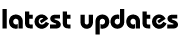# Physics :Heat and Work

Modern physics sees heat as energy collectively possessed by the particles making up a gas, liquid or solid. A body which possesses energy has the ability to do work. Work is done when a force (F) moves through a distance (d): W = F x d. If F is measured in newtons and d in metres, then W is measured in Nm, otherwise called joules.

THERMODYNAMICS

Thermodynamics is the study of the behaviour and properties of heat, energy and temperature within systems.

The first law of thermodynamics: The first law of thermodynamics states that the total amount of energy in any closed system always remains the same. In other words, energy is always conserved as it is transferred from one form to another.

The second law of thermodynamics: The second law of thermodynamics states that heat will always flow from a hotter object to a colder one, and not the other way round. It involves the term entropy. Entropy is a measure of the disorder of a system.

The third law of thermodynamics: The third law states that on approaching absolute zero, extracting energy from a system becomes increasingly harder. All bodies have thermal energy, or heat. Absolute zero is the theoretical point at which a body ceases to have any heat. This value is -273.15°C (-459.67°F) or 0°K (Kelvin). At this temperature, which is impossible to physically attain, the molecules in a body will cease to vibrate, and thus the body will have no internal energy.

## No comments

Be the first one to leave a comment.My Great Web page